Importance: Medium ✭✭
 Author(s): Jain, Kamal
 Subject: Graph Theory » Coloring » » Nowhere-zero flows
 Keywords: nowhere-zero flow
 Prize: none
 Posted by: mdevos on: March 7th, 2007
Conjecture   For every graphwithout a bridge, there is a flow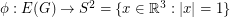.

Conjecture   There exists a map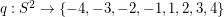so that antipodal points ofreceive opposite values, and so that any three points which are equidistant on a great circle have values which sum to zero.

The main interest in these two conjectures is that together they imply Tutte's 5-flow conjecture. This follows easily from the fact that the 5-flow conjecture can be reduced to cubic graphs without bridges, and for such a graph, the composition of the mapsand(given by the above conjectures) is a nowhere-zero 5-flow.

There are a couple of easy partial results toward the first conjecture which follow from well-known flow/cycle-cover results. First, Tutte showed that every graph with a nowhere-zero 4-flow has a list of three 2-flows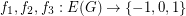so that every edge is in the support of exactly two of these flows. Combining these flows and normalizing appropriately gives an-flow. Bermond, Jackson, and Jaeger [BJJ] showed that every graph with no bridge has a list of seven 2-flows so that every edge is in the support of exactly four of these flows. Combining these and normalizing appropriately gives an-flow.

It seems likely that a graph has an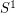-flow if and only if it has a nowhere-zero 3-flow. The "if" direction of this implication isn't hard to show and the "only if" direction looks quite possible.

A dual concept to that of a flow is that of a tension. Observe that a graphhas atension if and only if can be embedded in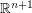so that all edges are unit length line segments. Such embeddings have received some attention over the years. In particular, there is considerable interest in finding the best possible upper bound on the chromatic number of graphs which embed inin this manner. This is Hadwinger-Nelson problem on coloring the plane.

## Bibliography

[BJJ] J.C. Bermond, B. Jackson, and F. Jaeger, Shortest covering of graphs with cycles, J. Combinatorial Theory Ser. B 35 (1983), 297-308. MRhref{0735197}

[T54] W.T. Tutte, A Contribution on the Theory of Chromatic Polynomials, Canad. J. Math. 6 (1954) 80-91. MathSciNet

[T66] W.T. Tutte, On the Algebraic Theory of Graph Colorings, J. Combinatorial Theory 1 (1966) 15-50. MathSciNet

* indicates original appearance(s) of problem.